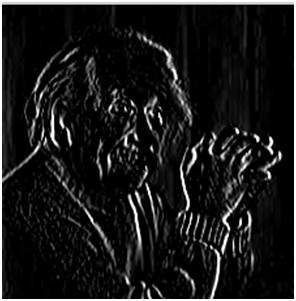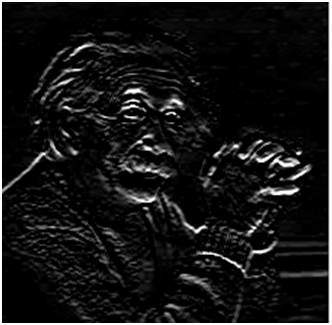# Prewitt Operator

Prewitt operator is used for edge detection in an image. It detects two types of edges

• Horizontal edges
• Vertical Edges

Edges are calculated by using difference between corresponding pixel intensities of an image. All the masks that are used for edge detection are also known as derivative masks. Because as we have stated many times before in this series of tutorials that image is also a signal so changes in a signal can only be calculated using differentiation. So that’s why these operators are also called as derivative operators or derivative masks.

All the derivative masks should have the following properties:

• Opposite sign should be present in the mask.
• Sum of mask should be equal to zero.
• More weight means more edge detection.

Prewitt operator provides us two masks one for detecting edges in horizontal direction and another for detecting edges in an vertical direction.

## Vertical direction

 -1 0 1 -1 0 1 -1 0 1

Above mask will find the edges in vertical direction and it is because the zeros column in the vertical direction. When you will convolve this mask on an image, it will give you the vertical edges in an image.

### How it works

When we apply this mask on the image it prominent vertical edges. It simply works like as first order derivate and calculates the difference of pixel intensities in a edge region. As the center column is of zero so it does not include the original values of an image but rather it calculates the difference of right and left pixel values around that edge. This increase the edge intensity and it become enhanced comparatively to the original image.

## Horizontal Direction

 -1 -1 -1 0 0 0 1 1 1

Above mask will find edges in horizontal direction and it is because that zeros column is in horizontal direction. When you will convolve this mask onto an image it would prominent horizontal edges in the image.

### How it works

Now it’s time to see these masks in action:

### Sample Image

Following is a sample picture on which we will apply above two masks one at time.After applying vertical mask on the above sample image, following image will be obtained. This image contains vertical edges. You can judge it more correctly by comparing with horizontal edges picture.